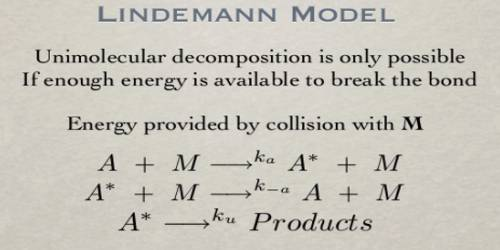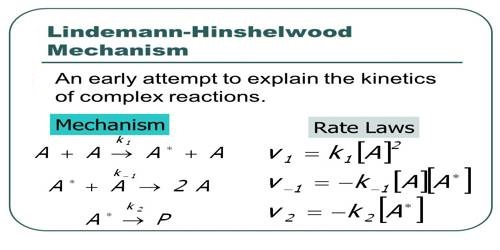# Unimolecular Reaction: Lindemann’s Mechanism

Unimolecular Reaction: Lindemann’s Mechanism

The collision theory gives a fairly satisfactory account of bimolecular reactions. The difficulty was, however, encountered in explaining the formation of the activated complex in the case of some of the reactions that were kinetically of first-order and apparently unimolecular.

The rate of formation of the active molecules by binary collisions should be proportional to n2, where n is the number of molecules per cm3. In a bimolecular reaction, the rate is also proportional to and second-order kinetics is followed. In a first-order reaction, however, the rate is proportional to n. In such reactions, the rate is not proportional to the rate of formation of active molecules. The question then arises as to how the molecules in such reactions acquire the necessary activation energy.

At one time it was seriously proposed that activation energy is acquired by absorption of radiation from the surroundings, but all attempts to detect such radiation were unsuccessful. The radiation hypothesis has, therefore, been rejected on valid grounds. F. A. Lindemann (1922) showed how activation by collision could lead to first-order kinetics for unimolecular reactions and also explain the breakdown of first-order rate law at low concentrations. He pointed out that molecules could be activated by collisions sub other molecules by transfer of energy, and after a molecule has acquired the necessary energy of activation it must remain at that energy state for a while before it can react. If, however, the activated molecule cannot have that ‘lifetime’ and undergoes collision, it would be deactivated. This necessarily implies the idea that activated molecules cannot cross over the energy barrier if they enter auto other collisions. Consequently, ‘activation by collision’ and ‘deactivation by collision’ are both possible. The idea can be mathematically treated as follows:

A + A ↔ k1 ↔ A* (k2 ↓ product) + A … … (1)

Where A* represents the activated molecule. As one can see (equation 1) the proposed mechanism also considers deactivation of A*. A Lindemann mechanism typically includes an activated reaction intermediate, labeled A* (where A can be any element or compound). The activated intermediate is produced from the reactants only after a sufficient activation energy is applied. It then either deactivates from A* back to A or reacts with another (dis)similar reagent to produce yet another reaction intermediate or the final product.Now the rate of activation is equal to k1 [A]2 and the rate of deactivation is k-1 [A*] [A]. The rate of reaction is, however, k2 [A*]. At steady state- d[A*]/dt = k1 [A]2 – k-1 [A*] [A] – k2 [A*] = 0

and, therefore, [A*] = k1 [A]2 / k-1 [A] + k2

or rate of reaction = k2 [A*] = k-1k-2 [A]2 / k-1 [A] + k2 … … … (2)

A limiting case may be considered.

Case: At sufficiently high pressure, k-1 [A] ˃˃ k2, then equation (2) may be written as-

rate = k-1k-2 [A]2 / k-1 [A] = Constant x [A]

The rate is proportional to the first power of concentration, i.e., the reaction is first order; that is if the rate of deactivation is sufficiently large, the reaction will follow a first-order rate law. This, of course, means that after activation there is the appreciable time lag before the molecules fall apart, and during this time lag, the activated molecules may undergo further collisions leading to deactivation.